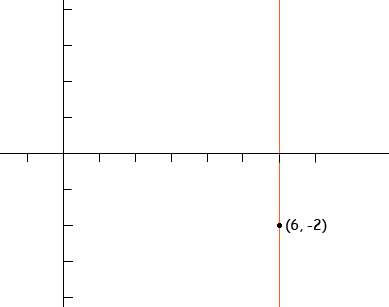SEARCH HOMEMath Central Quandaries & QueriesSubject: Writing the Equation of a Vertical Line passing (Point1,Point2) Name: Alex Who are you: Student How do I write an equation of a vertical line passing a coordinate of points? Such as: Write the equation of a vertical line passing through (6,-2)Hi Alex,

Think about the graph of the vertical line through (6, -2).The x-coordinate of every point on the graph is 6. Furthermore if a point in the plane is not on the graph then its x-coordinate is not 6. Hence a point (x,y) in the plane is on the graph if and only is x = 6. Thus the equation of the vertical line through (6, -2) is

x = 6.

PennyMath Central is supported by the University of Regina and The Pacific Institute for the Mathematical Sciences.